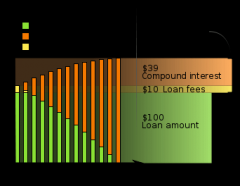# How Do You Calculate APR?APR is Annual Percentage Rate. The APR is the amount of interest that is normally charged on a loan, mortgage or a credit card. APR is calculated by the lender using a method of credit scoring and working out your ability to pay back the borrowed finance. For example, a low APR rate will most likely be offered to persons with a regular income and good credit history. If you have missed payments and perhaps only work on a temporary basis, your APR will be much higher.

The best way to find out the lowest APR you can get is to apply for finance to a few companies. You can then work out which is the best deal for you based on the offers you are given. Bear in mind that each time you apply a credit check will be carried out which will be recorded on your credit file also, so other companies can see what you have applied for in the past.
Take the "rate" and divide it by 100 to convert to decimal and then divide by the number of times it is compounded in a year.  Add 1 to that number and take it to the power of the number of times of compounding.  Subtract 1 from that nlumber anld multiply byl 100 to convert back to percentage..  E.g  loan rate = 3.65%  = .0365 in decimal form  divided by 365 (daily compounding)  = .0001  + 1 =1.0001  ^365 = 1.037172411    -1  = .037172411    X 100 = 3.7172411%
Loan £15000 interest rate 8.6%
It is kind of tricky to calculate APR and I have had a hard time trying to figure out how to do this, but the following article has helped me figure out what is and isn't included in calculating APR:

www.bankapedia.com
Calculating APR is an incredibly complex calculation and unless you are a math major at MIT, something you will probably need an APR calculator for. This site does a nice job of breaking APR down in layman's terms.
www.bankapedia.com

APR stands for Annual Percentage Rate, which is the official rate of borrowing or cost of debt. It takes into account the interest of the loan applied and also other miscellaneous charges. To be exact, it is how much the bank charges you for applying a loan.

To calculate APR for credit cards, you have to divide the finance charge in the balance sheet for your credit card over the debt owned to bank times 100% to get the APR rate.

To calculate APR for mortgage loans, it is much harder. It is better off using an online calculator to sort it off for you as it involved complex algorithms.